# 和屌丝一起学SWIFT-属性

[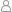孤狼 |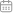2016-04-25 21:10:16 |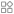Swift |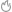2037°C |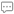0条评论 ]本教程仅用于初学SWIFT同学使用，内容由本人（孤狼）学习过程中笔记编写，本教程使用SWIFT版本为2.0。本教程内容可以自由转载，但必须同时附带本声明，或注明出处。PAEA.CN版权所有。

Hello，欢迎回到和屌丝一起学SWIFT系列教程中来，上节我们说了类和结构体，不知道大家有没有学会呢？它们可是很重要的哦，记得一定好好好复习哦．这节我们来一起学习一个和它们相关的东西－属性．这节也很重要，因为你以后会经常用到这些．

[存储属性]

``struct A{    var a1=0;    let a2=100;}var b1=A();print(b1.a2);   //输出"100"b1.a2=800;      //报错,因为a2属性使用的是let定义的常量无法修改let b2=A();b2.a1=800;      //报错,因为b2是一个常量,所以就算他的属性是变量,也无法修改.``

[延迟存储属性]

``class C{    lazy var str="我延迟了";}``

[计算属性]

TIP:get方法中最后一定要使用return来返回,否则报错.

``struct D{    var num=0;    var fun:Int{        get{            return num+10;        }        set(newNum){            num=newNum;        }    }}var d=D();print(d.fun);   //输出"10"d.num=10;       //通过直接修改方法设置print(d.fun);   //输出"20"d.fun=30;       //通过set方法设置print(d.fun);   //输出"40"``

[属性观察器]

``struct E{    var num=0{        willSet{            print(num);        }        didSet{            print(num);        }    }}var e=E();e.num=10;//输出:"0","10"``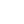赞赏一个鸡腿